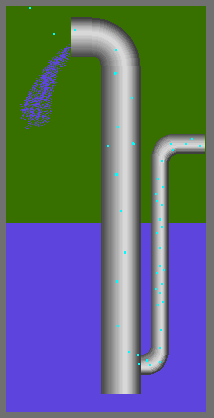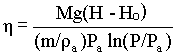### Air-lift Pump

Home -> Lecture Notes -> Fluid Mechanics -> Unit-V

Compressed gas is sometimes used for transferring liquid from one position to another in a chemical plant, but more particularly for emptying vessels. It is frequently more convenient to apply pressure by means of compressed gas rather than to install a pump, particularly when the liquid is corrosive or contains solids in suspension.

Several devices have been developed to eliminate the necessity for manual operation of valves, and the automatic acid elevator is an example of equipment incorporating such a device. The air-lift pump makes more efficient use of the compressed air and is used for pumping corrosive liquids. Although it is not extensively used in the chemical industry, it is used for pumping oil from wells.From hydrostatics, the pressure at the base of the column is obtained as,

where e is the volume fraction of air in the column of liquid of height H and r is the density of liquid.

If a mass (M) of liquid is raised through a net height (H-Ho) by a mass (m) of air, the net work done on the liquid is Mg(Ho-H).

If the pressure of the entering air is P, the net work done (W) by the air in expanding isothermally to atmospheric pressure Pa is given by:

W = ò PdV = -ò VdP

For isothermal process PV = constant, and V = nRT/P

Therefore,

W = nRTò dP/P

= PaVa ln(P/Pa)

where Pa is the atmospheric pressure and P is the inlet pressure of air.

substituting for Va in terms of mass(m) and density(ra) of air,

W = Pa(m/ra)ln(P/Pa)

The expansion will be almost exactly isothermal because of the intimate contact between the liquid and the air.

The efficiency (h ) of the pump is given by:If all losses in the operation of the pump were neglected, the pressure at the point of introduction of the compressed air would be equal to the atmospheric pressure together with the pressure rgHo (refer equn.1)

writing for atmospheric pressure in terms of head of liquid,

Pa = Harg and

P = (Ha + Ho)rgIt will be seen that mass of air required per mass of liquid raised, (m/M) decreases as Ho increases (i.e. submergence increase). If Ho is zero, (m/M) is infinite and therefore the pump will not work. A high submergence is therefore desirable.

The submergence, expressed as the ratio Ho/H, should vary from 0.66 for a lift of 20 feet to 0.41 for a lift of 500 feet. Thirty percent mechanical efficiency is usually obtained.

There are a number of important applications of the air-lift pump in the process industry due to its simplicity. It is particularly useful for handling radioactive materials as there are no mechanical parts in contact with the fluid, and the pump will operate virtually indefinitely without the need for maintenance which can prove very difficult when handling radioactive liquids.